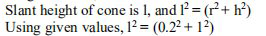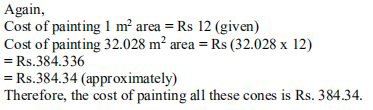# NCERT Solutions Class 9 Mathematics Solutions for Surface Areas and Volumes - Exercise 13.3 in Chapter 13 - Surface Areas and Volumes

Question 8 Surface Areas and Volumes - Exercise 13.3

A bus stop is barricaded from the remaining part of the road, by using 50 hollow cones

made of recycled cardboard. Each cone has a base diameter of 40 cm and a height of 1 m. If the

outer side of each of the cones is to be painted and the cost of painting is Rs. 12 per \mathbf{m}^{2}

what will be the cost of painting all these cones? (Use π = 3.14 and take √(1.04) = 1.02)

Given:

Radius of cone, r = diameter/2 = 40/2 cm = 20 cm = 0.2 m

Height of cone, h = 1 m= (1.04)

Or l = 1.02

The slant height of the cone is 1.02 m

Now,

CSA of each cone = πrl

= (3.14 x 0.2 x 1.02)

= 0.64056

CSA of 50 such cones = (50 x 0.64056) = 32.028

CSA of 50 such cones =

32.028 \mathrm{m}^{2}Video transcript
"hello students welcome to lito q a video session i am saf your math tutor and question for today is a bus stop is barricaded from the remaining part of the road by using 50 hollow phones made of recycled cardboard each phone has a base diameter of 40 centimeter and height 1 meter if the outer side of each of the cone is to be painted and the cost of painting is rupees 12 per meter square what will be the cost of painting all these cones use pi is equal to 3.14 and take root of 1.04 is equal to 1.02 let us see what is given given is the diameter that is 40 centimeter hence radius r can be given as 40 upon 2 half of the diameter is radius so 20 centimeter which can be written as 0.2 meter height is given 1 meter and slant height is not given so let us say slant height of the cone l and l will be equal to root of h square plus r square so root of 1 square plus 0.2 square and that is root of 1.04 in the question value of 1 root of 1.04 is 1.02 so our slant height is root of one point zero four that is one point zero two meter once you found the slant height you can easily found curve surface area of cone so curl surface area that is c as a of one cone that will be equal to pi into r into l pi value has to be taken as 3.14 radius 0.2 and slant height l that is 1.02 so this is the slant height l and here current surface area that is csa comes out to be for one cone it is 0.64056 unit is meter square now we can write it like this that current surface area of one cone is zero point six four zero five six meter square so what will be the curved surface area of 50 such cones because there are 50 holophones and that will be equal to 0.64056 into 50 and that is 32.028 meter square this is for the 50 such cones now it is given that the cost of painting is rupees 12 per meter square so for one meter square area cost is rupees 12 so how much will be the cost of 32.028 meter square area so for 32.028 meter square area your the cost will be 12 multiplied by 32.028 and that is rupees 384 point three three six rupees will be your cost this is the approximate cost that is 384 point three four that is approximate in rupees therefore we can write our statement in conclusion that the cost of painting all these cones that is 50 cones is rupees 384.34 so it is our final answer if you have any query you can drop it in our comment section and subscribe to ledo for more such q a thank you for watching "
Related Questions

Lido

Courses

Teachers

Book a Demo with us

Syllabus

Maths
CBSE
Maths
ICSE
Science
CBSE

Science
ICSE
English
CBSE
English
ICSE
Coding

Terms & Policies

Selina Question Bank

Maths
Physics
Biology

Allied Question Bank

Chemistry
Connect with us on social media!